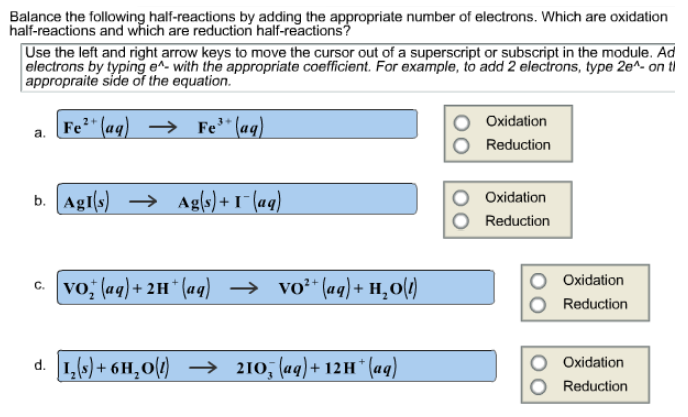# Problem: Balance the following half-reactions by adding the appropriate number of electrons. Which are oxidation half-reactions and which are reduction half-reactions ? Use the left and right arrow keys to move the cursor out of a superscript or subscript in the module. Add electrons by typing e- with the appropriate coefficient. For example, to add 2 electrons, type 2e- on the appropriate side of the equation. a. Fe2+ (aq) → Fe3+ (aq) b. AgI (s) → Ag (s) + I- (aq) c. VO2+ (aq) + 2H+ (aq) → VO2+ (aq) + H2O (l) d. I2 (s) + 6H2O (l) → 2IO3- (aq) + 12H+ (aq)

###### FREE Expert Solution
95% (327 ratings)###### Problem Details

Balance the following half-reactions by adding the appropriate number of electrons. Which are oxidation half-reactions and which are reduction half-reactions ?

Use the left and right arrow keys to move the cursor out of a superscript or subscript in the module. Add electrons by typing e- with the appropriate coefficient. For example, to add 2 electrons, type 2e- on the appropriate side of the equation.

a. Fe2+ (aq) → Fe3+ (aq)

b. AgI (s) → Ag (s) + I(aq)

c. VO2+ (aq) + 2H+ (aq) → VO2+ (aq) + H2O (l)

d. I2 (s) + 6H2O (l) → 2IO3- (aq) + 12H+ (aq)